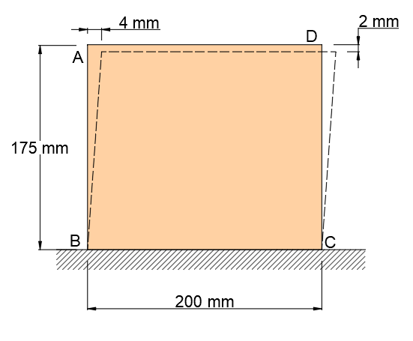# a. Determine the average normal strain of side AB of the plate. b. Determine the average...

## Question:

a. Determine the average normal strain of side AB of the plate.

b. Determine the average shearing strain at point A.## Normal Strain and Shear Strain :

The normal strain is obtained by dividing the change in length by the original length .Here the applied force is normal force

The shear strain is defined as the tangent of the angle of deformation .Deformation must in the direction parallel to shear force application.

Become a Study.com member to unlock this answer!

a) The normal strain in side AB can be found by dividing the change in length by the original length of side AB .

Given :

{eq}AB= 175 \ mm...Shear Strain: Definition & Equation

from

Chapter 2 / Lesson 10
114K

Shear strain is an important concept to know in engineering. Learn the definition of shear strain, discover the equation to calculate it, and see how to apply it using a specific example.